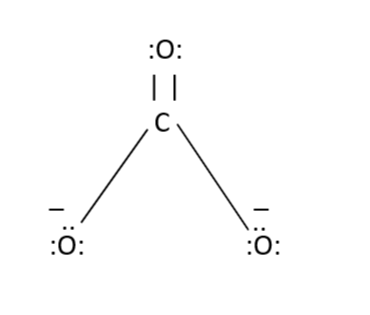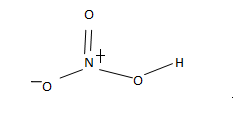QuestionAnswers

# What is the formal charge on atoms in carbonate and nitric acid?

Hint: The charge assigned to an atom in a molecule is known as formal charge and is calculated by the formula $FC = V - N - B/2$ .
Where V-> Number of valence electrons of an atom in its ground state.
N-> Number of nonbonding electrons
B-> The total number of electrons which are shared in covalent bond
Formal charge is calculated using $FC = V - N - B/2$
The carbonate ion $[C{O_3}^{2 - }]$ already has a charge of 2-So, the carbonate ion carbon atom is connected with two oxygen atoms with the help of single bonds and with one oxygen atom by double bond as shown above.
Now let us calculate the formal charge of the oxygen atom which is connected to the carbon atom with the help of double bond-
Here,
$V = 6$ (Since oxygen has 6 valence electrons- 2 in 2s subshell and 4 in 2p subshell)
$N = 4$ (You can count it from the figure, that there are 4 electrons which are not taking place in bonding)
$B = 4$ (A double bond between C and O which means 4 electrons)
On calculating we get-
$6 - 4 - 4/2 = 0 \\ \\$

Now we will calculate for the formal charge of oxygen atom with the single bond:
Here,
$V = 6$
$N = 6$ (You can SEE it from the figure itself)
$B = 2$ (As there is a single bond only)
$6 - 6 - 2/2 = - 1$
Now for Nitric acid i.e. $HN{O_3}$Here,
For the oxygen atom with the single bond
$V = 6$
$N = 6$
$B = 2$
$6 - 6 - 2/2 = - 1$
For oxygen atom with the double bond
$V = 6$
$N = 4$ (since two of oxygen electrons are used in bonding, 4 are left with it)
$B = 4$
$6 - 4 - 4/2 = 0$
For Nitrogen atom
$V = 5$ (since nitrogen atom has 5 valence electrons in its outermost shell)
$N = 0$
$B = 8$
$5 - 0 - 8/2 = 1$
Note:
Students must know the value of valence electrons for each atom.
Valence electrons are the electrons which are present in the outermost shell and can take part in chemical bonding.